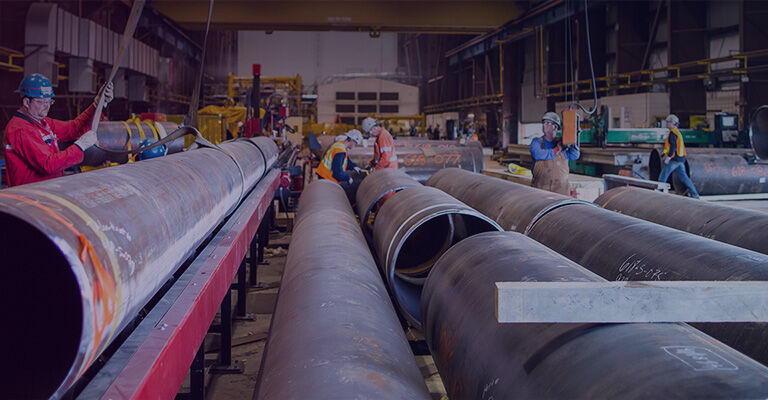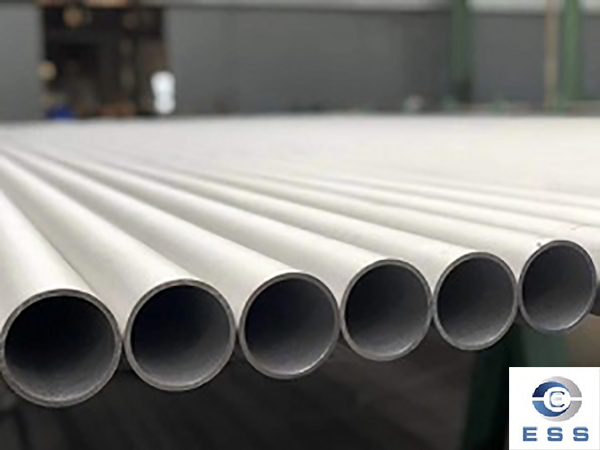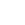## Company News## Company NewsYou are in :  Home  >  News  >  Company News

# Essential Tools and Formulas for Seamless Pipe Weight Calculation

Date:2023-06-01View:118Tags:Seamless Pipe,Seamless Pipe Weight Calculation
Essential Tools and Formulas for Seamless Pipe Weight Calculation

Seamless pipes are widely used in industries such as oil and gas, petrochemicals, power generation, and many others. The weight of seamless pipes is an important parameter that needs to be calculated accurately as it affects the transportation, installation, and other aspects of pipe handling. In this article, we will discuss the essential tools and formulas required for seamless pipe weight calculation.Tools Required for Seamless Pipe Weight Calculation

1.Pipe Weight Calculator
A pipe weight calculator is a tool that helps in calculating the weight of a seamless pipe based on its dimensions and material. There are many online pipe weight calculators available that can be used for this purpose. These calculators are easy to use and can provide accurate results.

2. Vernier Caliper
A vernier caliper is a tool that is used to measure the dimensions of a seamless pipe. It is a precision instrument that can measure the outside diameter, inside diameter, and wall thickness of a pipe accurately. This tool is essential for seamless pipe weight calculation as the dimensions of the pipe are required for the calculation.

3. Weighing Scale
A weighing scale is used to measure the weight of the seamless pipe accurately. This tool is essential for calculating the weight of the pipe as it provides the actual weight of the pipe that is required for the calculation.

Formulas for Seamless Pipe Weight Calculation

1.Weight Calculation Formula for Carbon Steel Pipes
The weight of a carbon steel seamless pipe can be calculated using the following formula:
W = 0.02466 x S x (D - S) x L

Where:W = Weight of the pipe in kg/meter
S = Wall thickness of the pipe in mm
D = Outside diameter of the pipe in mm
L = Length of the pipe in meter

2.Weight Calculation Formula for Stainless Steel Pipes
The weight of a stainless steel seamless pipe can be calculated using the following formula:
W = 0.02507 x S x (D - S) x L

Where:W = Weight of the pipe in kg/meter
S = Wall thickness of the pipe in mm
D = Outside diameter of the pipe in mm
L = Length of the pipe in meter

3.Weight Calculation Formula for Alloy Steel Pipes
The weight of an alloy steel seamless pipe can be calculated using the following formula:
W = 0.02507 x S x (D - S) x L x F

Where:W = Weight of the pipe in kg/meter
S = Wall thickness of the pipe in mm
D = Outside diameter of the pipe in mm
L = Length of the pipe in meter
F = Factor for alloy steel (The factor varies for different grades of alloy steel)

Conclusion

Seamless pipe weight calculation is an important aspect of pipe handling in various industries. The accuracy of the weight calculation is essential for the transportation, installation, and other aspects of pipe handling. The tools required for seamless pipe weight calculation include a pipe weight calculator, vernier caliper, and weighing scale. The formulas for seamless pipe weight calculation vary for different materials and can be calculated using the dimensions of the pipe. By using the essential tools and formulas discussed in this article, the weight of a seamless pipe can be calculated accurately.